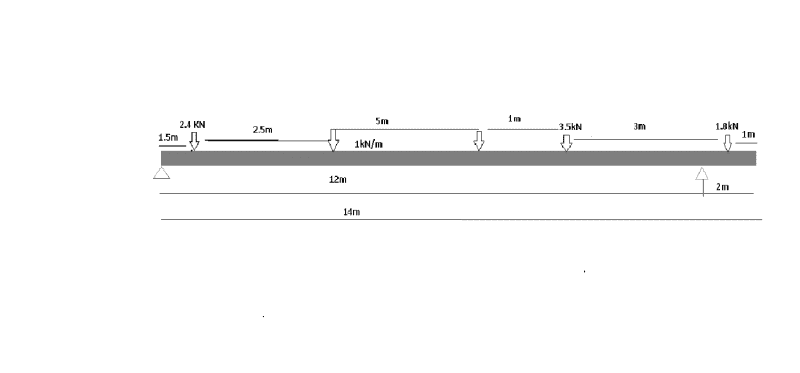# Determine the reaction forces at the supports

Hello, so I've been given this question and I get a different anwser then my friends. Can you check if what I wrote is right? If its wrong then can you tell me what have I done wrong? Sorry for the quality of the picture but my Paint skills are not so great :)I was measuring to the right support
RA is the left support, RB is the right support. Here is my calculations:
Sum of anticlockwise movement = Sum of clockwise movement
forces to the right support= 2.4 + 5 + 3.5 = 10.9kN
force x distance to the support= (3.5kN x 2m) + ( 5kN x 5.5m) + (2.4kN x 10.5m)=59.7kN
59.7kN=sum of clockwise movement
1.8kN + RB12 = 59.7kN
59.7-1.8=57.9kN
57.9/12=RB
RB=4.825
so RA= 59.7kN- 4.825
RA=54.875 kN

Last edited by a moderator:

gneill
Mentor
Hello MNWO. You should use the formatting template when you create a thread in the homework sections (in fact it's a requirement).

As it stands there's no clear statement of what the problem is: You haven't stated exactly what it is you hope to calculate. That statement should be given in the Problem Statement section of the template. You also need to define what the forces are that are indicated on your drawing; one of them is given as 1 kN/m. How are we to interpret that? As a distributed load? If so, how is it distributed?

Assuming that the beam is at static equilibrium, why don't you just set the moment equal to zero and sum the moments across the beam?

For instance, taking the moments about RA

∑Mra = 0 = -1.5m(2.4kN) + ..... you do the rest.

Where are you getting 3.5kN x 2m?

The 3.5kN is not a distributive force on your diagram, it's a concentrated force that is 10m from RA.

You cannot solve this problem by just summing the forces in the Y direction - you have two unknowns - you need two equations. Namely a moment equation and a sum of the forces equation, or two moment equations.

With your solution the beam would be accelerating in the Y-direction - making it into a dynamics problem.

Edit: by clockwise and anti-clockwise movements I'm assuming you mean moments in which case you're adding them up wrong. Moments depend on TWO tings, the force and the distance from the axis of rotation. Remember that torque is the cross product of the distance and the force T = R X F . You can't sum up the forces by themselves first. Remember your order of operations from math.

Hello MNWO. You should use the formatting template when you create a thread in the homework sections (in fact it's a requirement).

As it stands there's no clear statement of what the problem is: You haven't stated exactly what it is you hope to calculate. That statement should be given in the Problem Statement section of the template. You also need to define what the forces are that are indicated on your drawing; one of them is given as 1 kN/m. How are we to interpret that? As a distributed load? If so, how is it distributed?

I think the 1kN/m is distributed over the 5m that it is located under because the two arrows on either side don't have any loads on them. That's how I interpreted it. I'm pretty sure we're looking at a basic static equilibrium problem.

SteamKing
Staff Emeritus
Homework Helper
Hello, so I've been given this question and I get a different anwser then my friends. Can you check if what I wrote is right? If its wrong then can you tell me what have I done wrong? Sorry for the quality of the picture but my Paint skills are not so great :)
View attachment 76408

I was measuring to the right support
RA is the left support, RB is the right support. Here is my calculations:
Sum of anticlockwise movement = Sum of clockwise movement
forces to the right support= 2.4 + 5 + 3.5 = 10.9kN
force x distance to the support= (3.5kN x 2m) + ( 5kN x 5.5m) + (2.4kN x 10.5m)=59.7kN
59.7kN=sum of clockwise movement

You mean 'moment' here, rather than 'movement'. The units of moment are in kN-m and not kN.

You've taken the support at B as the reference in writing the moment equation above.
1.8kN + RB12 = 59.7kN
You can't use the reaction at B in this equation, because the moment @ RB = 0, since it is the refererence
59.7-1.8=57.9kN
57.9/12=RB
RB=4.825
so RA= 59.7kN- 4.825
RA=54.875 kN

You are subtracting forces from moments, which you can't do, because forces and moments have different units. You must add up all the known forces applied to the beam and use them to solve for the unknown reaction.